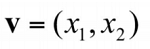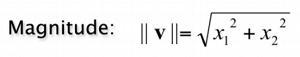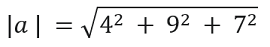# Vector Magnitude using Function | Linear Algebra using Python

Linear Algebra using Python: Here, we are going to learn about the Vector Magnitude using Function, python implementation of it.
Submitted by Anuj Singh, on May 12, 2020

Prerequisite: Linear Algebra | Defining a Vector

Linear algebra is the branch of mathematics concerning linear equations by using vector spaces and through matrices. In other words, a vector is a matrix in n-dimensional space with only one column. In the sequence of learning linear algebra using python, this is article 6. In linear algebra, the vector magnitude is given by:

In a scalar product, each component of the vector is multiplied by the same scalar value. As a result, the vector's length is increased by a scalar value.For example: Let a vector a = [4, 9, 7], then the magnitude of the vector is:The python code calculates the magnitude of the vector by defining a function. Why do we need to separately build a function? - This is helpful for the case when you have to find magnitudes of multiple vectors multiple times.

## Python code for Vector Magnitude using Function

```# Vectors in Linear Algebra Sequnce (7)
# Fuction defined for calculating magnitude
def magnitude(vec):
summ = 0
for i in range(len(vec)):
summ = vec[i]*vec[i] + summ
return pow(summ,0.5)

a = [2, 5, 2, 5, 14]
c = 3
b = []
print("Vector a = ", a)
summ = 0

print("Vector's magnitude = ", magnitude(a))
```

Output

```Vector a =  [2, 5, 2, 5, 14]
Vector's magnitude =  15.937377450509228
```

Preparation

What's New

Top Interview Coding Problems/Challenges!